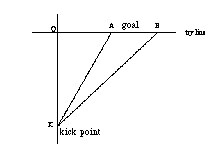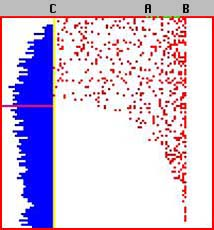# Modeling Rugby: Kick First, Generalize Later?

 URI WILENSKY Center for Connected Learning Northwestern University Annenberg Hall 311 2120 Campus Drive evanston, IL 60208 uriw@media.mit.edu 847-467-3818 Epistemology & Learning Group Learning & Common Sense Section The Media Laboratory Massachussets Institute of Technology 20 Ames Street Room E15-315 Cambridge, MA 02139 uriw@media.mit.edu

### Modeling Rugby: Kick First, Generalize Later?

At the NATO Advanced Research Workshop on Computers and Exploratory Learning (see diSessa, Hoyles and Noss, 1995) the organizers proposed several mathematical challenge problems. One problem that caught my attention was drawn from a British mathematics textbook and involved the game of rugby: (Italicized text is verbatim from the textbook, non-italicized comments in brackets are the author's).

In rugby, after a try has been scored, the scoring team has the opportunity to gain further points by "kicking a conversion." [i.e. Kicking the ball into the goal - the segment AB in the figure].The kick can be taken from anywhere on an imaginary line [line CK in the figure] that is perpendicular to the try line [line CB in the figure] and goes through the point that the try was scored [C in the figure]. Where should the kick be taken from to maximize the chance of a score? (In other words, where should we place the point K, so as to maximize the angle AKB?).The authors of the textbook intended for the problem to be solved as a calculus problem. One was to maximize the angle AKB subject to the constraints. Some of the people at the workshop solved the problem in this way. Others used plane geometry or dynamic geometry environments to come to a solution. Seeing through the lens of "object-based parallel modeling languages" such as StarLogo (Resnick, 1994; Wilensky, 1995), I saw it, not as a calculus problem, but rather as a problem in discrete probability. A colleague (M. Resnick) saw this as well and we decided to construct a solution using StarLogo.

We imagined several rugby players standing in each patch along the perpendicular line, CK, with each player kicking thousands of balls in random directions. To find the best kicking point, we simply needed to determine which rugby player scored the most conversions. It was quite easy to write the StarLogo program that would implement this strategy. The basic idea is to setup players at each patch on the kicking line. Let each player kick balls randomly. When a ball scores, update a counter in the patch from which it originated. When all balls have either scored or missed, reset the balls and kick again. At the end of the snapshot, I have included a listing of the StarLogo program (translated into the new version of Macintosh StarLogo). As in object-based parallel languages, the balls are kicked simultaneously and check themselves at each step to see whether they have scored or gone out of bounds.Thousands of kicked balls. To the left of the try line is a histogram of the successful kicks.

The technology of StarLogo facilitated our seeing the problem as amenable to probabilistic methods. Indeed, the most important piece of mathematics that can be garnered from this example is not the numerical solution to the particular problem, but the transformation of the problem from one of calculus or geometry to one of discrete probability. It is in making the connection between these different ways of seeing the problem, these different ways of measuring angles, that the powerful mathematics lies . The contribution of the StarLogo technology is in facilitating connections to stochastic mathematical processes whose relevance was much less obvious without such an object-based parallel modeling technology. This is a special case of the more general point that mathematics itself is a cognitive technology and the areas of mathematics we explore (or construct) are greatly constrained by the supporting technologies we possess. The application of probabilistic methods to the measurement of angles is not a natural operation without the availability of powerful computational tools. This lack of tools which allow intuitive probabilistic judgments is one important reason why probability has been a neglected area of mathematics education and why probabilistic thinking is so uncommon in our culture.

### Which solution is more general?

According to common mathematical wisdom, the StarLogo solution is not satisfying because it is less general, it gives us a numerical answer to a particular problem, not a general formula for line segment, CK, in terms of the variables CA and AB.

Note, however, that the notion of mathematical generality itself is relative both to the underlying supporting technologies, the potential contexts of application and the social purpose accorded the mathematics education.

In a computation rich world, is an algebraic formula more general than an algorithm? Is an analytic solution more general than a probabilistic approach?

Seeing this problem as a school mathematics problem (embedded in the social context of school mathematics) causes us to immediately ignore the real world conditions of the problem. We reflexively transform the problem from one about rugby to one about measuring angles. We implicitly assume that the kick is equally hard from any distance, in the real world an absurd assumption. We also assume that the grain of the grass is uniform and has no effect on the ball's trajectory and that the wind similarly has no effect. For this newly conceived, suitably transformed problem, the technology of plane geometry or calculus is adequate. These assumptions make the problem tractable using traditional school methods, but they also remove its connections from the world of our experience. The analytic solution is brittle, it works only under the idealized conditions of the problem. In contrast, the modeling solution can be easily modified to take account of differing conditions. We can experiment with the patches' grass rules letting them have different grains and seeing the differing results. We can introduce a wind of some velocity in some direction and see the results and we can introduce human factors such as the kicking ability of the player into the model.

### Is modeling enough?

By engaging in computational modeling -- this trialogue between the symbolism, the program output and the real world -- and, then, reflecting on the feedback obtained, learners can make meaningful connections. They can make both intra-mathematical connections -- connections within the sub-disciplines of mathematics such as calculus, geometry and probability -- as well as inter-domain connections -- connections between mathematical ways of knowing and everyday knowledge of sports, weather and human physiology.

It would be a mistake to interpret the above anecdote as demonstrating the superiority of object-based parallel modeling to traditional mathematical approaches. Indeed, used as another exercise in a traditional mathematics classroom, the StarLogo solution could facilitate inter-domain connections, but might serve to prevent making intra-mathematical connections. To facilitate these latter connections, modeling languages must be embedded in a "Connected Mathematics"-like environment (Wilensky, 1993; 1995), in which the pursuit of longer term investigations and wide connectivity are fostered and modeled. In the rugby example, many such avenues for connection are easily pursued. What are the level curves of constant "viewing angle" to the goal? What is the shape of these curves? What is their spacing, i.e. how fast does the viewing angle decline with distance from the goal? If kicking strength is to be modeled, what function governs the decay of the ball's velocity? What is the local effect of the grass grain and air resistance on the ball's trajectory? What probability distribution could be associated with a real kicker's kicking accuracy? By pursuing these questions and other questions of their own, learners can connect sub-disciplines of mathematics usually taught in isolation, allowing them to see and make mathematics not as a disconnected set of techniques to be memorized, but as a coherent and meaningful way of making sense of their experience.

### StarLogo Code Listing

As in object-based parallel languages, the procedures "kick" and "reset-balls" are executed at each clock tick by each ball kicked. The procedures "mon" and "update-stats" are executed once at each clock tick by the "observer" . Text after semi-colons are non-executable comments.

turtles-own [stopped scored orig-y px py] ;;; the balls' state variables

patches-own [pscored lang rang gang slope] ;;; the patches' state variables

globals [done curmax dist clock col ang ] ;;; global variables

;; rugby player kicks a ball, run this procedure forever

to kick

if stopped = 0 [fd 1] ;; at each clock tick the ball moves forward one unit

if out-of-bounds? [setstopped 1 stop] ;; no goal, try again

if goal? [setstopped 1 setscored scored + 1 tsetpscored-at (perp-line - xcor) (orig-y - 50)

(pscored-at (perp-line - xcor) (orig-y - 50)) + 1 stop]

;;; kick scored, increment the running total (pscored) for the patch from which the ball was kicked

end

;;; update the statistics and reset when needed, run this forever

to mon

update-stats ;; show a histogram of the number of scores from each patch on the kicking line

if done [stop-buttons show-results stop]

if ((total "stopped) = number) [reset-balls]

;;; if all the balls are stopped, set up new balls for the kickers

end

;; after all balls are determined, start over

to reset-balls

setclock clock + 1 ;;; update the number of times the players have kicked

setstopped 0 ;;;; all of the balls are back in play

setxy perp-line -50 + (who mod 100) ;;; each ball moves to its place on the kicking line

setorig-y ycor ;;; each ball remembers where it started

seth random 90 ;;; each ball gets a random heading

end

;;; creates the histogram of number of scores per kick site

to update-stats

setcurmax max "pscored

if pscored = curmax [psetdist (50 - ycor)]

graph-scores ;;;; draw the histogram

end

;;;; patch procedure to draw the histogram

to graph-scores

if (pc = blue) or (pc = pink) [setpc white] ;;; white out the histogram

if (xcor < perp-line) and ((perp-line - xcor) < ((pscored-at (perp-line - xcor) 0) * (perp-line + 50) / curmax)) [setpc blue] ;;; set the blue bars

if (xcor < perp-line) and ((pscored-at (perp-line - xcor) 0) = curmax) and ((perp-line - xcor) < ((pscored-at (perp-line - xcor) 0) * (perp-line + 50) / curmax)) [setpc pink] ;;;; color the maximum bar pink

end

; The following StarLogo code produces colorful curves of constant viewing angle:

;; calculate angle between patch and goal

to calc-gangle

setlang towards-patch left-goal 50 ;;; calculate angle with left goal post

setrang towards-patch right-goal 50 ;;; calculate angle with right goal post

setgang (rang - lang) mod 360 ;;;; calculate viewing angle

end

;;; draw a level curve of constant viewing angle

to level-curve :ang :col

if :ang = gang [setpc :col] ;;;; color yourself if your viewing angle is equal to the given angle

end

### References

diSessa, Hoyles and Noss (1995). Computers and Exploratory Learning. Springer.

Papert, (1996). An Exploration in the Space of Mathematics Educations. International Journal of Computers for Mathematical Learning, Vol. 1. No. 1. Kluwer Academic.

Resnick, M. (1994). Changing the Centralized Mind. in Technology Review . v97, n5.

Wilensky, U. (1995). Paradox, Programming and Learning Probability: A Case Study in a Connected Mathematics Framework, Journal of Mathematical Behavior, Vol. 14, No. 2.

Wilensky, U. (1993). Connected Mathematics: Building Concrete Relationships with Mathematical Knowledge. Doctoral dissertation. Cambridge, MA: MIT.